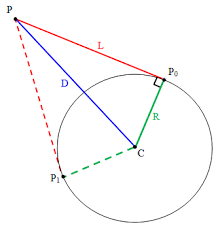Click to Chat

1800-1023-196

+91-120-4616500

CART 0

• 0

MY CART (5)

Use Coupon: CART20 and get 20% off on all online Study Material

ITEM
DETAILS
MRP
DISCOUNT
FINAL PRICE
Total Price: Rs.

There are no items in this cart.
Continue Shopping
        From a point on the line 4x-3y=6 , tangents aredrawn to the circle x2+y2-6x-4y+4=0 which make an angle of (tan)-1 24/7 between them. Find the co-ordinates of all such points and the equations of the tangents.
one year ago

							This problem looks pretty hard but can be simplified using transalation of axes to bring the circle’s center at the origin. We know that the circle’s center lies at (3, 2) and its radius is 3. Hence, we shift the origin to (3, 2) and the equations transform into the following form:x2+y2=3 – 4x-3y=0 –  Now, visualize a point on the line  extending two tangents on the circle  which makes an angle whose tangent is 24/7. Now (as in figure), angleP1PP2 is equally divided into CPP2 and CPP1 which can be equal to .(as tan must be positive, due to acuteness) Now we have found the angle CPP2 which can be used to find another equation of .  (radius=3 and length of tangent=S1) On equating the values of tan, we get another circle on which our required points lie...  and we already know  On solving, you get  Hence, you can now shift back the origin and find the equations of the pairs of tangents using the standard formula pretty easily. Thanks,Shukant Pal

one year ago
Think You Can Provide A Better Answer ?

## Other Related Questions on Analytical Geometry

View all Questions »### Course Features

• 731 Video Lectures
• Revision Notes
• Previous Year Papers
• Mind Map
• Study Planner
• NCERT Solutions
• Discussion Forum
• Test paper with Video Solution### Course Features

• 53 Video Lectures
• Revision Notes
• Test paper with Video Solution
• Mind Map
• Study Planner
• NCERT Solutions
• Discussion Forum
• Previous Year Exam Questions